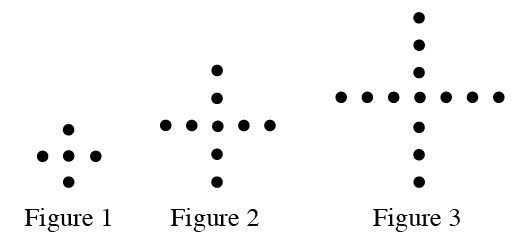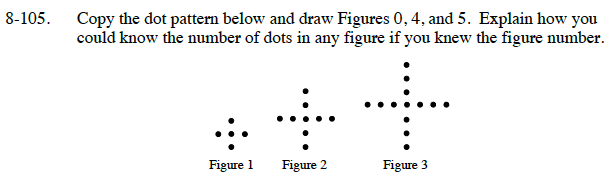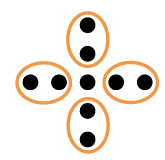Home > CC1 > Chapter 8 > Lesson 8.3.2 > Problem8-105

8-105.

Copy the dot pattern below and draw Figures 0, 4, and 5. Explain how you could know the number of dots in any figure if you knew the figure number. Homework Help ✎Could you write an expression to find the number of dots in a figure?

Look at Figure 2 to the right where 4 groups of 2 dots are circled and there is one dot left over.
An expression representing the number of dots in this figure would be 1 + 4x, where x is the figure number.
Use this to help you draw the next figures.